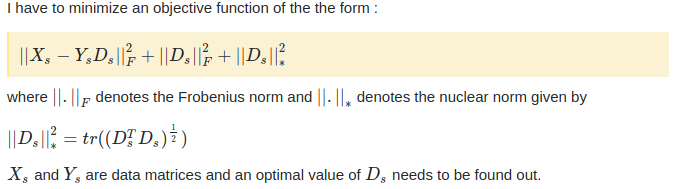# How to minimize an objective function containing frobenius and nuclear norms?subject to the constraint that square of Frobenius norm of Ds matrix has to be less than or equal to 1.

Currently I am using CVXPY library to solve the objective function. My code sample looks like

``````import cvxpy as cp
import numpy as np

np.random.seed(1)
Xs = np.random.randn(100,4096)
Ys = np.random.randn(100,300)

# Define and solve the CVXPY problem.
Ds = cp.Variable(shape=(300,4096))

lamda1 = 1

obj = cp.Minimize(cp.square(cp.norm(Xs - (Ys*Ds),'fro'))
+ lamda1*cp.norm(Ds,'nuc')) constraints = [cp.square(cp.norm(Ds,'fro')) <= 1]

prob = cp.Problem(obj, constraints)
prob.solve(solver=cp.SCS, verbose=True)
``````

The console gives an error that

Solver ‘SCS’ failed. Try another solver or solve with verbose=True for more information. Try recentering the problem data around 0 and rescaling to reduce the dynamic range.

I event tried to experiment with different solvers like cp.ECOS but they do not optimize the function.

Any suggestions ?

This forum addresses CVX, not CVXPY, which is a different tool. Please post CVXPY questions at https://groups.google.com/forum/#!forum/cvxpy

However, I’ll give you some freebie advice, try using Mosek as solver if you can (not sure about problem size, though). Maybe also think about which norms you want or need squared, and don’t square them unless necessary (certainly not needed for norm fro constraint).

Here is what this would look like in CVX. Use square_pos around norm_nuc if you want that squared. I didn’t square the norm fro in the constraint, which (squaring) serves no purpose (rather, apply sqrt to RHS, which in this case remains 1).

``````Xs = randn(100,4096);
Ys = randn(100,300);
lambda=1;
cvx_begin
variable Ds(300,4096)
minimize(square_pos(norm(Xs - (Ys*Ds),'fro')) + lambda*norm_nuc(Ds))
norm(Ds,'fro') <= 1
cvx_end
``````

It may take a while to run, given its size, and that `norm_nuc` makes it into an SDP.